International
Tables for
Crystallography
Volume C
Mathematical, physical and chemical tables
Edited by E. Prince

International Tables for Crystallography (2006). Vol. C, ch. 8.3, pp. 698-701

## Section 8.3.2. Stereochemically restrained least-squares refinement

E. Prince,a L. W. Fingerb and J. H. Konnertc

aNIST Center for Neutron Research, National Institute of Standards and Technology, Gaithersburg, MD 20899, USA,bGeophysical Laboratory, Carnegie Institution of Washington, 5251 Broad Branch Road NW, Washington, DC 20015-1305, USA, and cLaboratory for the Structure of Matter, Code 6030, Naval Research Laboratory, Washington, DC 20375-5000, USA

### 8.3.2. Stereochemically restrained least-squares refinement

| top | pdf |

The precision with which an approximately correct model can be refined to describe the atomic structure of a crystal depends on the ability of the model to represent the atomic distributions and on the quality of the observational data being fitted with the model. In addition, although the structure can in principle be determined by a well chosen data set only a little larger than the number of parameters to be determined (Section 8.4.4), in practice, with a nonlinear model as complex as that for a macromolecular crystal, it is necessary for the parameters defining the model to be very much over-determined by the observations. For well ordered crystals of small- and intermediate-sized molecules, it is usually possible to measure a hundred or more independent Bragg reflections for each atom in the asymmetric unit. When the model contains three position parameters and six atomic displacement parameters for each atom, the over-determinacy ratio is still greater than ten to one. In such instances, each model parameter can usually be quite well determined, and will provide an accurate representation of the average structure in the crystal, except in regions where ellipsoids are not adequate descriptions of the atomic distributions. This contrasts sharply with studies of biological macromolecules, in which positional disorder and thermal motion in large regions, if not the entire molecule, often limit the number of independent reflections in the data set to fewer than the number of parameters necessary to define the distributions of individual atoms. This problem may be overcome either by reducing the number of parameters describing the model or by increasing the number of independent observations. Both approaches utilize knowledge of stereochemistry.

A great deal of geometrical information with which an accurate model must be consistent is available at the onset of a refinement. The connectivity of the atoms is generally known, either from the approximately correct Fourier maps of the electron density obtained from a trial structure determination or from sequencing studies of the molecules. Quite tight bounds are placed on local geometry by the accumulating body of information concerning bond lengths, bond angles, group planarity, and conformational preferences in torsion angles. Additional knowledge concerns van der Waals contact potential functions and hydrogen-bonding properties, and displacement factors must also be correlated in a manner consistent with the known geometry. In Section 8.3.1, we discuss the use of constraints to introduce this stereochemical knowledge. In this section, we discuss a technique that introduces the stereochemical conditions as additional observational equations (Waser, 1963). This method differs from the other in that information is introduced in the form of distributions about mean values rather than as rigidly fixed geometries. The parameters are restrained to fall within energetically permissible bounds.

#### 8.3.2.1. Stereochemical constraints as observational equations

| top | pdf |

As described in Section 8.1.2, given a set of observations,, that can be described by model functions, Mi(x), where x is the vector of model parameters, we seek to find x for which the sum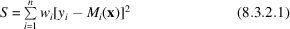is minimum. For restrained refinement, S is composed of several classes of observational equations, including, in addition to the ones for structure factors, equations for interatomic distances, planar groups and displacement factors.

Structure factors yield terms in the sum of the form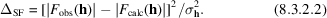The distances between bonded atoms and between next-nearest-neighbour atoms may be used to require bonded distances and angles to fall within acceptable ranges. This gives terms of the form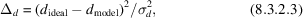where σd is the standard deviation of an empirically determined distribution of values for distances of that type. Groups of atoms may be restrained to be near a common plane by terms of the form (Schomaker, Waser, Marsh & Bergman, 1959)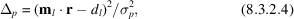where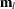and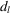are parameters of the plane, σp is again an empirically determined standard deviation, and · indicates the scalar product.

If a molecule undergoes thermal oscillation, the displacement parameters of individual atoms that are stereochemically related must be correlated. These parameters may be required to be consistent with the known stereochemistry by assuming a model that gives a distribution function for the interatomic distances in terms of the individual atom parameters and then restraining the variance of that distribution function to a suitably small value. The variation with time of the distances between covalently bonded atoms can be no greater than a few hundredths of an ångström. Therefore, the thermal displacements of bonded atoms should be very similar along the bond direction, but they may be more dissimilar perpendicular to the bond. If we make the assumption that the atom with a broader distribution in a given direction is `riding' on the atom with the narrower distribution, the variance of the interatomic distance parallel to a vector v making an angle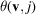with the direction of bond j is (Konnert & Hendrickson, 1980)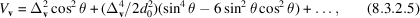where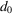is the normal distance for that type of bond,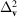=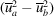, and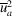and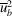are the mean square displacements parallel to v of atom a and atom b, respectively. The restraint terms then have the form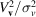. For isotropic displacement factors, these terms take the particularly simple form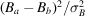, but with the disadvantage that, when isotropic displacement parameters are used, the displacements cannot be suitably restrained along the bonds and perpendicular to the bonds simultaneously.

Several additional types of restraint term have proved useful in restraining the coordinates for the mean positions of atoms in macromolecules. Among these are terms representing nonbonded contacts, torsion angles, handedness around chiral centres, and noncrystallographic symmetry (Hendrickson & Konnert, 1980; Jack & Levitt, 1978; Hendrickson, 1985). Contacts between nonbonded atoms are important for determining the conformations of folded chain molecules. They may be described by a potential function that is strongly repulsive when the interatomic distance is less than some minimum value, but only weakly attractive, so that it can be neglected in practice, when the distance is greater than that value. This leads to terms of the form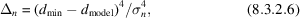which are included only when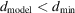. Macromolecules usually gain flexibility by relatively unrestricted rotation about single bonds. There are, nevertheless, significant restrictions on these torsion angles, which may, therefore, be restrained by terms of the form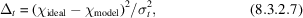where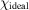and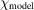are dihedral angles between planar groups at opposite ends of the bond.

Interatomic distances are independent of the handedness of an enantiomorphous group. If rc is the position vector of a central atom and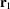,, and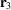are the positions of three atoms bonded to it, such that the four atoms are not coplanar, the chiral volume is defined by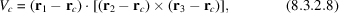where × indicates the vector product. The chiral volume may be either positive or negative, depending on the handedness of the group. It may be restrained by including terms of the form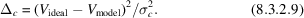Table 8.3.2.1gives ideal coordinates, in an orthonormal coordinate system measured in Å, of various groups that are commonly found in proteins. The ideal conformations of pairs of amino acid residues, from which the ideal values to be used in restraint terms of various types may be determined, are constructed by combining the coordinates of the individual groups. For example, consider a dipeptide composed of glycine and alanine joined by a trans peptide link, giving the moleculeThe origin is placed at each of the Cα positions in turn, and interatomic distances to nearest and next-nearest neighbours are computed. Planar groups and possible nonbonded contacts are identified, and torsion angles and chiral volumes for chiral centres are computed. Table 8.3.2.2is a summary of the restraint information for this simple molecule. In order to incorporate this information in the refinement, these ideal values are combined with suitable weights. Table 8.3.2.3gives values of the standard deviations of the various types of constraint relation that have been found (Hendrickson, 1985) to give good results in practice.

 Table 8.3.2.1| top | pdf | Coordinates of atoms (in Å) in standard groups appearing in polypeptides and proteins; restraint relations may be determined from these coordinates using methods described by Hendrickson (1985)
 Main chain, links and terminal groups.
 Main N 1.20134 0.84658 0.00000 Cα 0.00000 0.00000 0.00000 C −1.25029 0.88107 0.00000 O −2.18525 0.66029 0.78409 C terminal N 1.20006 0.84799 0.00000 Cα 0.00000 0.00000 0.00000 C −1.26095 0.86727 0.00000 O −2.32397 0.27288 −0.29188 Ot −1.15186 2.04837 0.35987 N amino terminal N 1.20134 0.84658 0.00000 Cα 0.00000 0.00000 0.00000 C −1.25029 0.88107 0.00000 O −2.18525 0.66029 −0.78409 N formyl terminal N 1.19423 0.82137 0.00000 Cα 0.00000 0.00000 0.00000 C −1.24896 0.88255 0.00000 O −2.10649 0.78632 −0.90439 Ot 2.46193 −0.77877 −0.93569 Ct 2.33913 0.39064 −0.53355 N acetyl terminal N 1.19423 0.82137 0.00000 Cα 0.00000 0.00000 0.00000 C −1.24896 0.88255 0.00000 O −2.10649 0.78632 −0.90439 Ot 2.46193 −0.77877 −0.93569 Ct1 2.33913 0.39064 −0.53355 Ct2 3.44659 1.39160 −0.63532 trans peptide link Cα 0.00000 0.00000 0.00000 C 0.57800 1.41700 0.00000 O 1.80400 1.60700 0.00001 N −0.33500 2.37000 0.00000 Cα 0.00000 3.80100 0.00000 cis peptide link Cα 0.00000 0.00000 0.00000 C 1.30900 0.79200 0.00000 O 2.38500 0.17600 0.00000 N 1.23500 2.11000 0.00000 Cα 0.00000 2.90700 0.00000 trans proline link Cα 0.00000 0.00000 0.00000 C 0.57800 1.41700 0.00000 O 1.80400 1.60700 0.00001 N −0.33500 2.37000 0.00000 Cα 0.00000 3.80100 0.00000 Cδ −1.80000 2.19600 0.00000 cis proline link Cα 0.00000 0.00000 0.00000 C 1.30900 0.79200 0.00000 O 2.38500 0.17600 0.00000 N 1.23500 2.11000 0.00000 Cα 0.00000 2.90700 0.00000 Cδ 2.45500 2.93900 0.00000
 Side chains for amino acids.
 Ala A Cβ 0.02022 −0.92681 1.20938 Arg R Cβ −0.02207 −0.93780 1.20831 Cγ −0.09067 −0.23808 2.55932 Cδ −0.79074 −1.07410 3.57563 N−0.76228 −0.46664 4.89930 Cζ −1.57539 −0.83569 5.89157 Nη1 −2.60422 −1.65104 5.68019 Nη2 −1.38328 −1.38328 7.11065 Asn N Cβ 0.04600 −1.02794 1.12104 Cγ −0.15292 −0.42844 2.50080 Oδ1 −0.39364 0.78048 2.63809 Nδ2 −0.06382 −1.27086 3.52863 Asp D Cβ 0.04600 −1.02794 1.12104 Cγ −0.15292 −0.42844 2.50080 Oδ1 −0.39364 0.78048 2.63809 Oδ2 −0.06930 −1.21904 3.46540 Cys C Cβ 0.01317 −0.95892 1.18266 Sγ −0.07941 −0.15367 2.80168 Gln Q Cβ −0.01691 −0.98634 1.16423 Cγ −0.08291 −0.32584 2.52866 Cδ −0.20841 −1.31760 3.65937 O1 −0.48899 −2.49684 3.46331 N2 −0.00450 −0.81846 4.87646 Glu E Cβ −0.06551 −0.87677 1.25157 Cγ 1.15947 −1.71468 1.59818 Cδ 1.40807 −2.90920 0.72611 O1 0.92644 −3.06007 −0.38343 O2 2.16269 −3.74330 1.27140 Gly G (no nonhydrogen atoms) His H Cβ −0.06434 −0.96857 1.20324 Cγ −0.52019 −0.29684 2.46369 Nδ1 0.26457 0.53405 3.22184 C1 −0.46699 1.05500 4.19371 N2 −1.69370 0.59727 4.09040 Cδ2 −1.75570 −0.25685 3.02097 Ile I Cβ 0.03196 −0.97649 1.23019 Cγ1 −0.83268 −2.22363 0.92046 Cγ2 −0.39832 −0.28853 2.54980 Cδ1 −0.77555 −3.32741 2.01167 Leu L Cβ 0.09835 −0.94411 1.20341 Cγ −0.96072 −2.02814 1.32143 Cδ1 −0.89548 −2.98661 0.13861 Cδ2 −0.73340 −2.79002 2.62540 Lys K Cβ −0.03606 −0.92129 1.21541 Cγ 1.19773 −1.81387 1.35938 Cδ 1.05466 −2.77178 2.53242 C2.34215 −3.51295 2.82637 Nζ 2.16781 −4.42240 3.98733 Met M Cβ 0.02044 −0.96506 1.17716 Cγ −1.00916 −2.05384 1.00286 Sδ −0.77961 −3.24454 2.37236 C−2.08622 −4.42220 1.97795 Phe F Cβ 0.00662 −1.03603 1.11081 Cγ 0.03254 −0.49711 2.50951 Cδ1 −1.15813 −0.12084 3.13467 C1 −1.15720 0.38038 4.42732 Cζ 0.05385 0.51332 5.11032 C2 1.26137 0.11613 4.50975 Cδ2 1.23668 −0.38351 3.20288 Pro P Cβ 0.12372 −0.78264 1.31393 Cγ 0.89489 0.13845 2.22063 Cδ 1.87411 0.86170 1.30572 Ser S Cβ −0.00255 −0.96014 1.17670 Oγ −0.19791 −0.28358 2.40542 Thr T Cβ −0.00660 −0.98712 1.23470 Oγ1 0.04119 −0.14519 2.43011 Cγ2 1.12889 −2.01366 1.21493 Trp W Cβ 0.02501 −0.98461 1.16268 Cγ 0.03297 −0.36560 2.51660 Cδ1 −1.03107 0.15011 3.20411 N1 −0.62445 0.62417 4.42903 C2 0.72100 0.41985 4.55667 Cζ2 1.57452 0.72329 5.60758 Cη2 2.91029 0.38415 5.45120 Cη3 3.37037 −0.23008 4.28944 C3 2.51952 −0.53303 3.24549 Cδ2 1.17472 −0.20516 3.37412 Tyr Y Cβ 0.00470 −0.95328 1.20778 Cγ −0.18427 −0.27254 2.54372 Cδ1 0.89731 0.26132 3.25049 C1 0.72371 0.85064 4.50059 Cζ −0.54776 0.88971 5.06861 C2 −1.63905 0.38287 4.37622 Cδ2 −1.44975 −0.19374 3.12415 Oη −0.76405 1.40409 6.31652 Val V Cβ 0.05260 −0.99339 1.17429 Cγ1 −0.13288 −0.31545 2.52668 Cγ2 −0.94265 −2.12930 0.99811
 Table 8.3.2.2| top | pdf | Ideal values for distances (Å), torsion angles (°), etc. for a glycine–alanine dipeptide with a trans peptide bond; distance type 1 is a bond, type 2 a next-nearest-neighbour distance involving a bond angle
 Interatomic distances.
Number   DistanceType
1 N(1) to C(1)α 1.470 1
2 Cα(1) to C(1) 1.530 1
3 C(1) to O(1) 1.240 1
4 N(1) to C(1) 2.452 2
5 C(1)α to O(1) 2.414 2
6 N(2) to C(2)α 1.469 1
7 C(2)α to C(2) 1.530 1
8 C(2) to O(2) 1.252 1
9 N(2) to C(2) 2.461 2
10 C(2)α to O(2) 2.358 2
11 C(2)β to C(2)α 1.524 1
12 C(2)β to C(2) 2.515 2
13 C(2)β to N(2) 2.450 2
14 C(2) to O(2)t 1.240 1
15 O(2) to O(2)t 2.225 2
16 C(2)α to O(2)t 2.377 2
17 N(2) to C(1) 1.320 1
18 N(2) to O(1) 2.271 2
19 N(2) to C(1)α 2.394 2
20 C(2)α to C(1) 2.453 2
 Planar groups.
 1 CTRM C(2)α C(2) O(2) O(2) 2 LINK C(1)α C(1) O(1) N(2) C(2)α
 Chiral centres.
Central atom   Chiral volume (Å3)
1 Ala C(2)α N(2) C(2) C(2)β 2.492
 Possible nonbonded contacts.
Number   Distance
1 N(1) to O(1) 3.050
2 N(2) to O(2) 3.050
3 O(2) to C(2)β 3.350
4 N(2) to O(2)t 3.050
5 O(2)t to C(2)β 3.350
 Torsion angles.
 N(1) C(1)α C(1) N(2) 0 C(1)α C(1) N(2) C(2)α 180 C(1) N(2) C(2)α C(2) 0 N(2) C(2)α C(2) O(2)t 0
 Table 8.3.2.3| top | pdf | Typical values of standard deviations for use in determining weights in restrained refinement of protein structures (after Hendrickson, 1985)
 Interatomic distances Nearest neighbour (bond) σd = 0.02 Å Next-nearest neighbour (angle) 0.03 Å Intraplanar distance 0.05 Å Hydrogen bond or metal coordination 0.05 Å Planar groups Deviation from plane σp = 0.02 Å Chiral centres Chiral volume σc = 0.15 Å3 Nonbonded contacts Interatomic distance σn = 0.50 Å Torsion angles Specified (e.g. helix ϕ and ψ) σt = 15° Planar group 3° Staggered 15°
 Thermal parameters Anisotropic Isotropic Main-chain neighbour σv = 0.05 Å σB = 1.0 Å2 Main-chain second neighbour 0.10 Å 1.5 Å2 Side-chain neighbour 0.05 Å 1.5 Å2 Side-chain second neighbour 0.10 Å 2.0 Å2

Even for a small protein, the normal-equations matrix may contain several million elements. When stereochemical restraint relations are used, however, the matrix elements are not equally important, and many may be neglected. Convergence and stability properties can be preserved when only those elements that are different from zero for the stereochemical restraint information are retained. The number of these elements increases linearly with the number of atoms, and is typically less than 1% of the total in the matrix, so that sparse-matrix methods (Section 8.1.5) can be used. The method of conjugate gradients (Hestenes & Stiefel, 1952; Konnert, 1976; Rae, 1978) is particularly suitable for the efficient use of restrained-parameter least squares.

### References

Hendrickson, W. A. (1985). Stereochemically restrained refinement of macromolecular structures. Methods in enzymology, Vol. 115. Diffraction methods for biological macromolecules, Part B, edited by H. W. Wyckoff, C. H. W. Hirs & S. N. Timasheff, pp. 252–270. New York: Academic Press.
Hendrickson, W. A. & Konnert, J. H. (1980). Incorporation of stereochemical information into crystallographic refinement. Computing in crystallography, edited by R. Diamond, S. Ramaseshan & D. Venkatesan, pp. 13.01–13.26. Bangalore: Indian Academy of Sciences.
Hestenes, M. & Stiefel, E. (1952). Methods of conjugate gradients for solving linear systems. J. Res. Natl Bur. Stand. 49, 409–436.
Jack, A. & Levitt, M. (1978). Refinement of large structures by simultaneous minimization of energy and R factor. Acta Cryst. A34, 931–935.
Konnert, J. H. (1976). A restrained-parameter structure-factor least-squares refinement procedure for large asymmetric units. Acta Cryst. A32, 614–617.
Konnert, J. H. & Hendrickson, W. A. (1980). A restrained parameter thermal-factor refinement procedure. Acta Cryst. A36, 344–350.
Rae, A. D. (1978). An optimized conjugate gradient solution for least-squares equations. Acta Cryst. A34, 578–582.
Schomaker, V., Waser, J., Marsh, R. E. & Bergman, G. (1959). To fit a plane or a line to a set of points by least squares. Acta Cryst. 12, 600–604.
Waser, J. (1963). Least-squares refinement with subsidiary conditions. Acta Cryst. 16, 1091–1094.